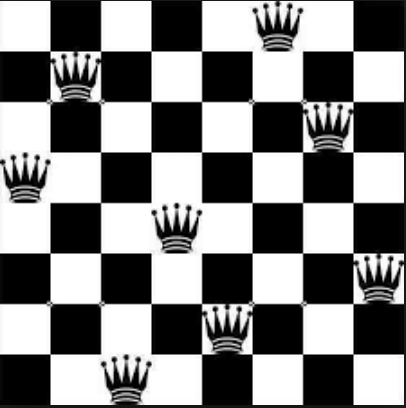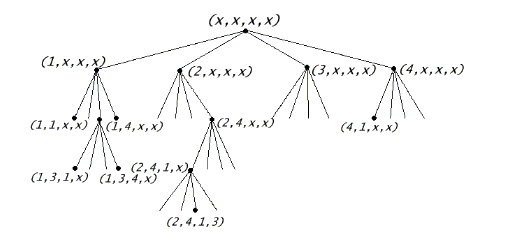1

SICP lec6a : 流 I part2 - 八皇后 (回溯搜索)“如何能夠在8×8的西洋棋棋盤上放置八個皇后，任兩個皇后都不能處於同一條橫行、縱行或斜線上。”

`(defn safe? [row col queens-position])`跳脫時間的方法

• 假設已有 k 列前(0 ~ k-1列)的所有解法，要繼續擴充到k列。
• 對k列的每個位置都用`safe?`做過濾。

(網路上抄的答案 kerker)

``````(defn safe?
"Check if queen in last row is safe"
[positions]
(let [[queen-pos & left] positions
k (count positions)
diags-up (map - left (range 1 (inc k)))
diags-down (map + left (range 1 (inc k)))]
(empty? (filter (partial = queen-pos) (concat diags-up diags-down left)))))
``````
``````(defn queens [board-size]
((fn queen-cols [k]
(if (zero? k)
(list empty-board)
(for [rest-of-queens (queen-cols (dec k))
new-row (range 1 (inc board-size))
:let [new-cols (cons new-row rest-of-queens)]
:when (safe? new-cols)]
new-cols)))
board-size))
``````

`rest-of-queens` -> k列前 所有可行的位置
`new-row` -> 新列的每一個element
`:let ... :when` -> 當通過`safe?`時 執行 `(cons new-row rest-of-queens)`